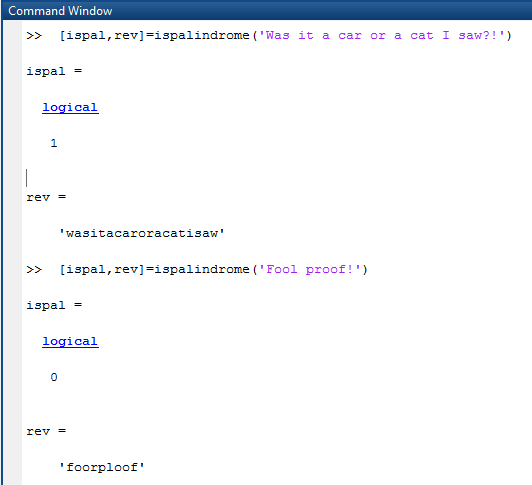# Answered! In matlab Write a function ispalindrome that returns whether a given input string is a palindrome and also its reversal (strip…

In matlab

Write a function ispalindrome that returns whether a given input string is a palindrome and also its reversal (strip out any non-alphabetical characters). A palindrome is a string that is the same as its reverse. e.g., “Was it a car or a cat I saw?!” is a palindrome because you get the same string when you reverse the order of the characters. The first thing you do with the input is to strip out any non-alphabetical characters and convert any upper case letters to lower case. So, “Was it a car or a cat I saw?!” will become “wasitacaroracatisaw”.

Don't use plagiarized sources. Get Your Custom Essay on
Answered! In matlab Write a function ispalindrome that returns whether a given input string is a palindrome and also its reversal (strip…
GET AN ESSAY WRITTEN FOR YOU FROM AS LOW AS \$13/PAGE

Example:

>> [ispal,rev]=ispalindrome(‘Was it a car or a cat I saw?!’)

ispal =
logical
1

rev =
‘wasitacaroracatisaw’
>> [ispal,rev]=ispalindrome(‘Fool proof!’)

ispal =
logical
0

rev =
‘foorploof’

function [ispal,rev]=ispalindrome(string)

len=length(string);
string=lower(string);
k=1;
for i=1:len
if double(string(i))>122||double(string(i))<97
continue
end
newString(k)=string(i);
k=k+1;
end
len2=length(newString);
for j=1:len2
rev(j)=newString(len2+1-j);
end
if rev==newString
ispal=true;
else
ispal=false;
end